×

Search anything:

# Three Way Partitioning

#### Algorithms Interview Problems on ArrayGet this book -> Problems on Array: For Interviews and Competitive Programming

In this article, we have explored Three Way Partitioning technique which is used in Three Partition Quicksort and Dutch National Flag Algorithm.

1. The Problem
2. Solving the Problem
3. The Algorithm
4. Implementation of the solution
5. Time and Space Complexity
6. Applications of Three Way Partitioning

Pre-requisites:

## The Problem

In this problem, an array is given along with a range consisting of two values, a low value (l) and a high value (h). The objective is to sort the given array in such a manner that three partitions can be obtained consisting of the following:

1. Values less than l.
2. Values between l and h.
3. Values greater than h.

Note that in this problem, the elements in each partition need not be sorted, they can appear in any order. Also note that the values l and h need not be present in the array.

Example

Consider the array [6, 9, 11, 3, 8, 5, 19, 21] with l = 7, and h = 12. Then, [3, 5, 6, 8, 9, 11, 19, 21] as well as [ 5, 3, 6, 9, 8, 11, 21, 19] are both correct solutions to three way partitioning.

## Solving the Problem

A solution that comes directly to mind on looking at this problem is that of sorting the given array. In a sorted array, elements less than l, elements between l and h, and elements greater than h will obviously be partitioned, and hence we are done. The average time complexity of such sorting algorithms shall be no less than O(nlogn).

However, one can note here that sorting the array is not the best possible solution, since it performs a lot of unnecessary comparisons and reordering of elements.

This calls for a more efficient solution, in which the elements in each partition need not be sorted.

## The Algorithm

One possible way of solving the given problem is by traversing the array and swapping elements in such a way that elements less than l get swapped towards the left, and those greater than h get swapped towards the right. For this, we follow the given algorithm:

1. Initialize two elements begin and end as the first and last positions of the array respectively.
2. Loop through the array with the counter variable i and do the following until i is greater than end.
1. If the value at the ith position is greater than h, then swap the element in the ith position with that in position end, and then decrement end.
2. Else if the value at the ith position is less than l, then swap the element in the ith with that in the position begin, and then increment begin as well as i.
3. Else if the value at the ith position lies between l and h, then increment the counter variable i.

Solving the Example

We shall now attempt to solve our earlier example using the algorithm. The array is given as follows: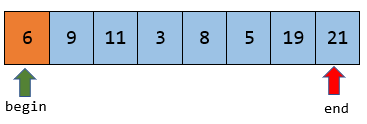Here, l = 7, and h = 12. We initialize begin and end as the first and last positions respectively. We start with the control variable i = 0.

Now, 6 is less than l, and hence, we swap it with the element at position begin, which in this case happens to be the same. We increment begin as well as i.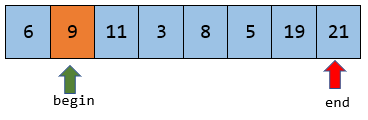Now, 9 is neither less than 7 nor greater than 12. Hence we increment i. Not that begin does not get incremented in this case.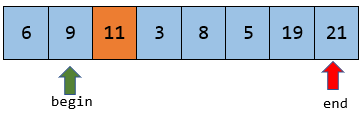As in the previous case, 11 lies between l and h, and hence we increment i.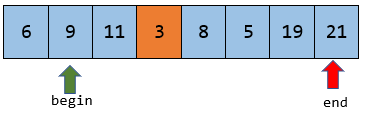Now, since 3 is less than l, we swap 3 and the element at position begin, which is 9. Then we increment both begin and i.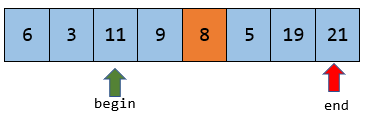Since 8 lies between 7 ans 12, we increment i.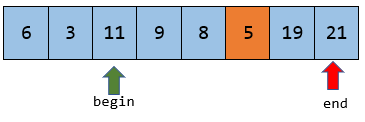Now, since 5 is less than l, we swap 5 and 11, and increment begin along with i.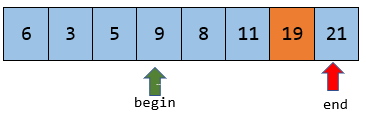Note now that 19 is greater than 12, and hence we swap it with the element at position end, and decrement end. Note that we do not increment i in this scenario.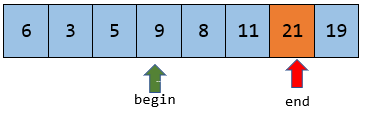Since 21 is greater than 12, we swap it with the element at position end, which is itself, and then decrement end. Now, since end is less than i, we end the loop, and we can observe that we have obtained a three-way partitioned array.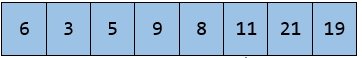## Implementation of the solution

Here, we show the implementation of the solution using C++.

``````#include<iostream>
using namespace std;

void TWP(int arr[], int n, int l, int h)
{
int begin = 0, end = n-1;
for(int i = 0; i <= end; i++)
{
if(arr[i] < l)
{
swap(arr[i], arr[begin]);
begin++;
}
else if(arr[i] > h)
{
swap(arr[i], arr[end]);
i--;
end--;
}
}
}

int main()
{
int arr[] = {6, 9, 11, 3, 8, 5, 19, 21};
int n = 8;
TWP(arr, n, 7, 12);
for(int i = 0; i < n; i++)
cout<<arr[i]<<endl;
}
``````

## Time and Space Complexity

Since we traverse over the elements of the array, and since the for loop shall run atmost n times, the time complexity of this solution is given by O(n). Since this solution does not use any extra memory, the space complexity is given by O(1).

## Applications of Three Way Partitioning

1. Three Partition Quicksort

Conventional quicksort picks a pivot element and partitions the array using this element. This process of partitioning continues until the array gets sorted.

Quicksort can also be performed using three way partitioning, if instead of one pivot element, two are picked, and the array is split into three partitions in each iteration.

The time complexity of quicksort using three way partitioning will be O(nlog3n), which is slightly better than the conventional solution, which has a time complexity of O(nlog2n).

2. Dutch National Flag Algorithm

In the Dutch National Flag Problem, the objective is to sort the given set of balls of three colors (red, blue, and white), such that balls of the same color come together.

To solve this problem using three- way partioning, we give values 0, 1, and 2 to the three colors. Then, we perform three way partitioning with l = h = 1. Hence, we obtain an array with different colors in each partition.

With this article at OpenGenus, you must have the complete idea of Three Way Partitioning.#### Aravind Mohandas

Aravind Mohandas is a Master's student at Indian Institute of Technology, Ropar with B.Sc in Computer Science from Christ University, Bangalore. He is an intern at OpenGenus.

Three Way Partitioning